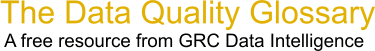Choose Index below for a list of all words and phrases defined in this glossary.

# Y=f(X)

index | Index

## Y=f(X) - definition(s)

Y=f(X) - In this equation X represents the input of the process and Y the output of the procees and f the function of the variable X.

Y is the dependent output variable of a process. It is used to monitor a process to see if it is out of control, or if symptoms are developing within a process. It is a function of the Xs that contribute to the process. Once quantified through Design of Experiment, a transfer function Y=f(X) can be developed to define the relationship of elements and help control a process.

Y is the output measure, such as process cycle time or customer satisfaction. f is the letter representing "function" (what the value(s) of X(s) does/do for Y (the output). X(s) is/are any process input(s) (variables) having assigned or inherent values(s) that is/are involved in producing the output.

For example, if you call your major department store to ask a question, the ability to have your question answered (Y) is a function (f) of the wait time, the number of people answering the phones, the time it takes to talk with the representative, the representative's knowledge, etc. All of these X's can be defined, measured and improved.

[Category=Data Quality ]

Source: iSixSigma, 05 March 2011 10:00:06, http://www.isixsigma.com/index.php?option=com_glossaryData Quality Glossary.  A free resource from GRC Data Intelligence. For comments, questions or feedback: dqglossary@grcdi.nl